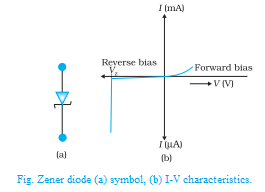# Zener diode

Zener diode is a reverse biased heavily doped semiconductor (silicon or germanium) PN junction diode, which is operated exclusively in the breakdown region.

The symbol of a Zener diode is shown in Fig. For normal operation of a Zener diode, in breakdown region, the current through the diode should be limited by an external circuit. Hence the power dissipated across the junction is within its power-handling capacity. Unless this precaution is observed, a large current will destroy the diode.Zener diode Symbol

The V-I characteristic curve for the Zener diode is shown in Fig. It can be seen from the figure that, as the reverse voltage applied to the PN junction is increased, at a particular voltage, the current increases enormously from its normal cut off value. This voltage is called Zener voltage or breakdown voltage (VZ).

## Zener diode as voltage regulator

To maintain a constant voltage across the load, even if the input voltage or load current varies, voltage regulation is to be made. A Zener diode working in the breakdown region can act as a voltage regulator.

The circuit in which a Zener diode is used for maintaining a constant voltage across the load RL is shown in Fig. The Zener diode in reverse biased condition is connected in parallel with the load RL. Let Vdc be the unregulated dc voltage and VZ be Zener voltage (regulated output voltage). RS is the current limiting resistor. It is chosen in such a way that the diode operates in the breakdown region.Zener Diode as DC voltage regulator

In spite of changes in the load current or in the input voltage, the Zener diode maintains a constant voltage across the load. The action of the circuit can be explained as given below:

Load current varies, the input voltage is constant: Let us consider that the load current increases. Zener current hence decreases, and the current through the resistance RS is a constant.

The output voltage is VZ = Vdc – IRS, since the total current I remains constant, the output voltage remains constant.

Input voltage varies: Let us consider that the input voltage Vdc increases. Now the current through Zener increases and the voltage drop across Rs increases in such a way that the load voltage remains the same. Thus the Zener diode acts as a voltage regulator.